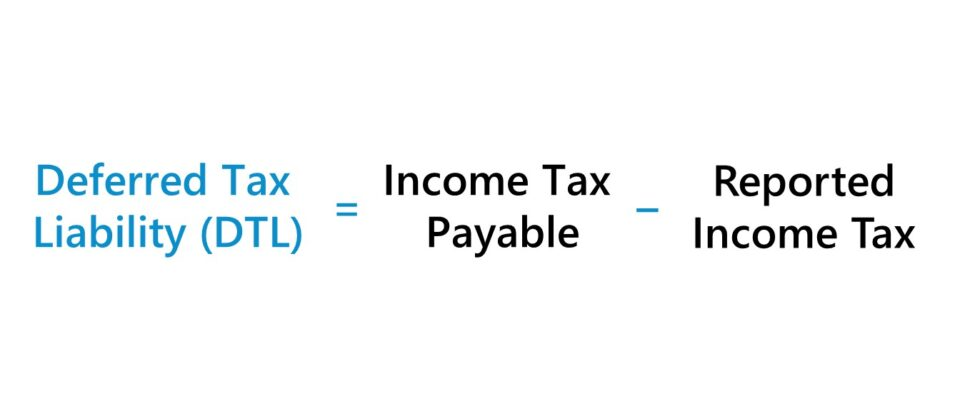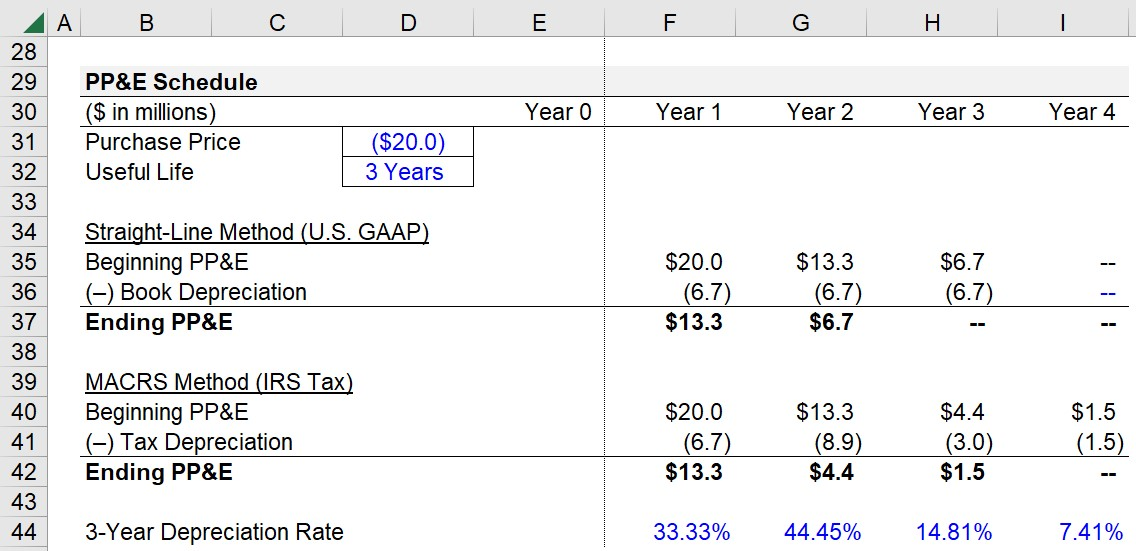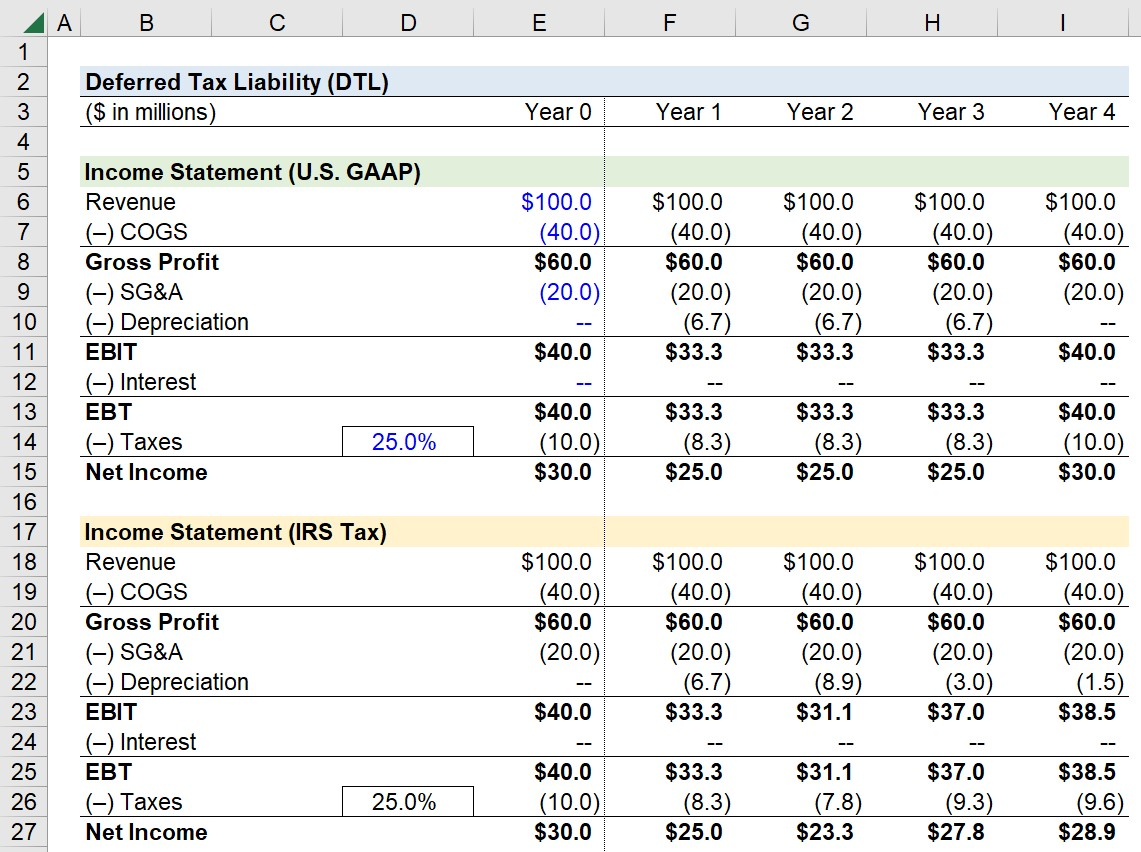Welcome to Wall Street Prep! Use code at checkout for 15% off.# Deferred Tax Liability (DTL)

Guide to Understanding the Deferred Tax Liability (DTL) Concept## What Creates a Deferred Tax Liability? (Step-by-Step)

A deferred tax liability, or “DTL”, is created when the income tax expense recorded on a company’s income statement prepared under GAAP accounting standards is different from the actual amount paid to the Internal Revenue Service (IRS).

The temporary timing mismatch between the recognized tax expense between GAAP and IRS accounting is treated as a liability because fewer taxes were actually paid to the IRS than the amount reported on the income statement.

Since the deferred tax liability is required to be paid soon in the near future, the tax account is categorized as a liability, i.e. the DTL represents a future obligation that must be fulfilled (“cash outflow”).

The discrepancy between the tax expense on the GAAP-based income statement and the actual taxes paid to the IRS is temporary and should gradually unwind to a balance of zero.

## Deferred Tax Liability Example: Depreciation (Book vs. Tax)

Most often, the reason that a deferred tax liability (DTL) arises is tied to a company’s depreciation recognition policies.

• Depreciation Expense→ Depreciation is an accounting concept where the cost of a capital expenditure—i.e. the purchase of a fixed asset (PP&E)—is recognized across its useful life assumption, rather than recognizing the entire expenditure during the period it was incurred.
• Useful Life → The useful life of fixed assets (PP&E) is the estimated time in which the fixed asset is anticipated to continue providing economic value to the company.
• Depreciation Method → The depreciation method describes the distribution of expense recognition across the fixed asset’s useful life, i.e. the allocation of the capital expenditure (Capex). For example, the depreciation expense is equal in all periods under straight-line depreciation, whereas more of the total expense is concentrated in the earlier periods in accelerated depreciation (e.g. MACRS, double declining method).

Companies have the discretion to utilize either straight-line depreciation or accelerated depreciation for GAAP reporting purposes.

Suppose a company decides to use straight-line depreciation but the depreciation method used for tax purposes is on an accelerated basis. In that case, the depreciation expense for tax purposes is greater in the earlier stages of the fixed asset’s useful life than under straight-line depreciation (and less in the later stages).

The cumulative depreciation recognized is equivalent, irrespective of whichever method is followed. Hence, the DTL is a temporary timing difference that’ll unwind over the course of the asset’s useful life because the depreciation expense used for tax reporting—upon reaching an inflection point—starts to reverse and reduce below the amount reported under GAAP until reaching a balance of zero.

## Deferred Tax Liability Formula (DTL)

The formula used to calculate the deferred tax liability (DTL) is as follows.

Deferred Tax Liability (DTL) = Income Tax Payable Reported Income Tax

Going off the prior depreciation example, the deferred tax liability (DTL) recorded on the balance sheet is calculated as the difference between the value of PP&E under book accounting and tax accounting in each period multiplied by the tax rate.

Deferred Tax Liability (DTL) = (Book PP&E Value Tax PP&E Value) × Tax Rate %

## Deferred Tax Liability vs. Deferred Tax Asset

• Deferred Tax Liability (DTL) → To reiterate from the earlier section, a deferred tax liability emerges from the company paying less in taxes to the IRS than the tax recorded on its income statement (GAAP)
• Deferred Tax Asset (DTA) → On the other hand, a deferred tax asset is created when the company pays more in taxes to the IRS than the tax expense recognized on its income statement (via GAAP). DTAs can be related to changes in revenue recognition and expense recognition policies, as well as from net operating losses (NOLs).

## Deferred Tax Liability Calculator (DTL) — Excel Model Template

We’ll now move to a modeling exercise, which you can access by filling out the form below.Submitting...

## Step 1. Deferred Tax Liability Exercise Assumptions

Suppose we’re tasked with preparing the income statement of a company for U.S. GAAP accounting purposes and tax purposes.

Our company uses straight-line depreciation for GAAP reporting purposes and MACRS for tax purposes.

The following is the company’s income statement data as of Year 0:

• Revenue = \$100 million
• (–) COGS = (40 million)
• Gross Profit = \$60 million
• (–) SG&A = (20 million)
• (–) Depreciation = 0 million
• EBIT = 40 million
• (–) Interest = 0 million
• EBT = \$40 million
• (–) Taxes @ 25% = (10 million)
• Net Income = \$30 million

For the rest of the forecast period, all of our assumptions—excluding “Depreciation” and “Taxes”—will be set equal to our assumptions in Year 0 and remain constant (i.e. “straight-lined”).

## Step 2. PP&E Schedule and Depreciation Calculation (Straight-Line vs. MACRS)

In the next step, we’ll calculate the depreciation expense using the straight-line method and the MACRS method.

The cost of the PP&E purchase will be assumed to be \$20 million with a useful life of 3 years and a salvage value of zero.

• Purchase Price = \$20 million
• Useful Life = 3 Years

Under the straight-line method, the depreciation expense will be \$6.7 million per year.

We’ll now calculate the depreciation expense per year under MACRS using the following list for reference.

3-Year MACRS Depreciation Rate (%)

• Year 1: 33.33%
• Year 2: 44.45%
• Year 3: 14.81%
• Year 4: 7.41%

Note: Under MACRS depreciation, the rate is one year longer than the asset’s classification.

Each year, we’ll multiply the applicable accelerated depreciation rate by the PP&E purchase price.

• MACRS Depreciation, Year 1 = \$6.7 million
• MACRS Depreciation, Year 2 = \$8.9 million
• MACRS Depreciation, Year 3 = \$3.0 million
• MACRS Depreciation, Year 4 = \$1.5 million## Step 3. Income Statement (U.S. GAAP vs. IRS Tax)

We’ll now link our depreciation expense values to our book and tax income statements.

Since we have our calculations in place, our tax expense should update once we input our depreciation values.## Step 4. Deferred Tax Liability Calculation Analysis

Our two income statements prepared under book and tax purposes illustrate how a deferred tax liability can be created.

Early on, there is a temporary timing difference resulting in lower payments to the IRS compared to the amount recorded for book purposes. But soon after, the deferred tax liability (DTL) reverses course as the tax payments to the IRS become higher to compensate for the discrepancy.

In conclusion, we’ll confirm the cumulative depreciation expense is \$35 million while the total cumulative tax expense is \$20 million under both book and tax accounting.Step-by-Step Online Course

### Everything You Need To Master Financial Modeling

Enroll in The Premium Package: Learn Financial Statement Modeling, DCF, M&A, LBO and Comps. The same training program used at top investment banks.

Inline Feedbacks